BAŞKENT ÜNİVERSİTESİ
Bilgi Teknolojileri ve Uygulamaları Koordinatörlüğü

Sample Questions

There will be an online exam including 25 multiple choice test items as shown below;

Note: There will be no lab exam. If student has a previous experience in using MS-Office programs in computer, she/he will be able to answer questions.

Sample Questions

Which of the following expressions is incorrect?

• Data and instructions in the computer are kept and processed as numbers.
• Computers use only two numbers: 0 and 1.
• Computer data kept as 8 bit series are called byte.
• 1 bit refers to one letter or character.
• Data in computer is carried out on metal lines called as data lines.

Which of the following buttons is used for line spacing in MS-Word?

•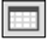•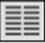•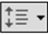•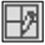•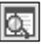Which of the followings cannot be performed when adding a photo album in Powerpoint?

• Determining number of photos in slides
• Making pictures black or white
• Change the order of photos

Which of the following formulas is used to get the sum between the cells A3 and A9 in MS-Excel?

• =SUM(A3;A9)
• =SUM(A3:A9)
• =SUM(A2*A10)
• =SUM(A3=A9)
• =SUM(A3_A9)

Which of the below fields can be identified as primary key on the same table in MS-Access?

• ID Number
• Name
• Surname
• Department
• Faculty